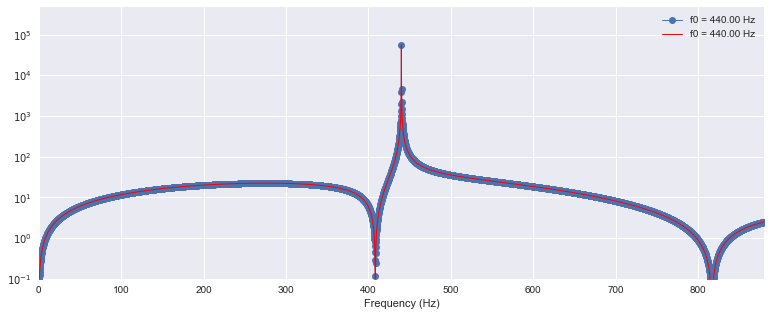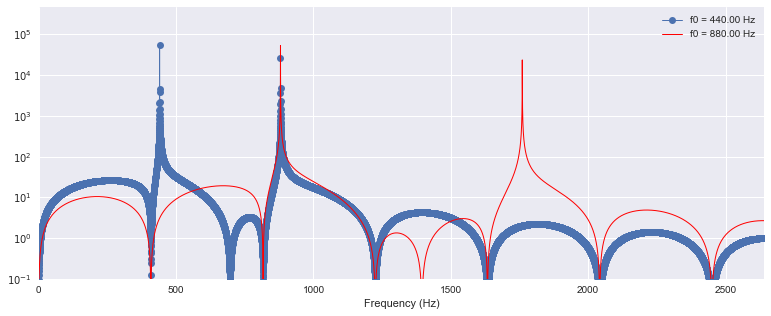In :
%matplotlib inline
import seaborn
import scipy, scipy.signal, IPython.display as ipd, matplotlib.pyplot as plt
plt.rcParams['figure.figsize'] = (13, 5)


# Tuning SystemsÂ¶

## IntroductionÂ¶

### Tuning SystemsÂ¶

In twelve-tone equal temperament (Wikipedia), all twelve semitones within the octave have the same width. With this tuning system, expressed as a frequency ratio, the interval of one semitone is $2^{1/12}$. Expressed in cents, this same interval is defined to be 100 cents. Therefore, the octave has 1200 cents.

In just intonation (Wikipedia), the frequency ratio is expressed as a fraction between two small integers, e.g. 3:2, 4:3. As a result, the higher harmonic partials between two notes will overlap, resulting in a consonant interval that is pleasing to the ear. In 5-limit just tuning, these fractions are expressed with prime factors no larger than 5, i.e. {2, 3, 5}. In 7-limit just tuning, these fractions are expressed with prime factors no larger than 7, i.e. {2, 3, 5, 7}. For example, 7:4 is a 7-limit interval, but it is not a 5-limit interval.

In Pythagorean tuning (Wikipedia), every frequency ratio is based upon the ratio 3:2. To find that ratio, from one note in the interval, step around the Circle of Fifths until you reach the other note in the interval, multiplying (if stepping forward) or dividing (if stepping backward) by 3/2 with each step. Finally, multiply or divide by 2 enough times to return to the octave of interest. Pythagorean tuning can also be considered 3-limit just tuning since every ratio only uses prime factors no greater than 3.

### How To Use This NotebookÂ¶

In the examples below, listen to each interval, and compare intervals both visually and aurally across tuning systems. In some tuning systems, the upper harmonics do not align. In such cases, try to listen for dissonance and beat frequencies. Then compare them to the tuning systems where the harmonics align perfectly.

### Notebook SetupÂ¶

Global parameters for this notebook:

In :
T = 5     # duration in seconds
sr = 22050  # sampling rate in Hertz
fcutoff = 4000 # frequency cutoff for filter


Functions used to create the sounds and figures:

In :
def simulate_tone(f0, harmonics=None):
"""Returns a tone with a specified fundamental frequency and harmonic amplitudes."""
if harmonics is None:
harmonics = 
t = scipy.linspace(0, T, T*sr, endpoint=False)
x = sum(v*scipy.sin(2*scipy.pi*i*f0*t) for i, v in enumerate(harmonics, 1))
return x

In :
def filter_tone(x, fcutoff=None):
"""Return a low-pass filtered signal."""
if fcutoff is None:
fcutoff = sr/2.0
h = scipy.signal.firwin(55, 2*float(fcutoff)/sr)
y = scipy.convolve(x, h)
return y

In :
def make_double_stop(ratio, f0=440, harmonics=None):
"""Listen to two tones played simultaneously, and plot the tones' spectra."""
if harmonics is None:
harmonics = 
f1 = ratio*f0

# Generate both tones.
x0 = filter_tone(simulate_tone(f0, harmonics=harmonics), fcutoff=fcutoff)
x1 = filter_tone(simulate_tone(f1, harmonics=harmonics), fcutoff=fcutoff)

# Add both tones, and normalize.
y = x0 + x1
y = 0.5*y/y.max()

# Generate both spectra.
X0 = scipy.fft(x0)
X1 = scipy.fft(x1)

# Create frequency variable.
N = len(X0)
f = scipy.linspace(0, sr, N, endpoint=False)

# Plot spectrum of both notes.
plt.semilogy(f, abs(X0), marker='o', linewidth=1)
plt.semilogy(f, abs(X1), color='r', linewidth=1)

plt.xlim(xmin=0, xmax=(len(harmonics)+1)*(f1 if ratio > 1 else f0))
plt.ylim(ymin=0.1)

plt.xlabel('Frequency (Hz)')
plt.legend(('f0 = %.2f Hz' % f0, 'f0 = %.2f Hz' % f1))

# Output audio widget.
print 'ratio between notes:', ratio
print 'difference in cents:', scipy.log2(ratio)*1200
return ipd.Audio(y, rate=sr)


## UnisonÂ¶

Within each section below, the intervals are provided in order of interval width from lowest to highest.

In :
make_double_stop(1)

ratio between notes: 1
difference in cents: 0.0

Out:## OctavesÂ¶

In :
harmonics = [1.0, 0.5]


Just intonation or equal temperament, 12 semitones, 1200 cents:

In :
make_double_stop(2, harmonics=harmonics)

ratio between notes: 2
difference in cents: 1200.0

Out:Pythagorean tuning, twelve steps forward on the Circle of Fifths. In Pythagorean tuning, multiply the fundamental frequency by 3/2 twelve times, and then divide by two enough times to return to the octave of interest:

$$\left( \frac{3}{2} \right)^{12} \left( \frac{1}{2} \right)^6 = \frac{531441}{262144} \approx 2.0273$$
In :
make_double_stop(531441.0/262144, harmonics=harmonics)

ratio between notes: 2.02728652954
difference in cents: 1223.46001038

Out: